## October 5, 2015

### Configurations of Lines and Models of Lie Algebras (Part 2)

#### Posted by John BaezTo get deeper into this paper:

we should think about the 24-cell, the $\mathrm{D}_4$ Dynkin diagram, and the Lie algebra $\mathfrak{so}(8)$ that it describes. After all, its this stuff that underlies the octonions, which in turn underlies the exceptional Lie algebras, which are the main subject of Manivel’s paper.

Remember that the root lattice of $\mathfrak{so}(2n)$ is called the $\mathrm{D}_n$ lattice: it consists of vectors in $\mathbb{R}^n$ with integer entries that sum to an even number.

I’m interested in $\mathfrak{so}(8)$ so I’m interested in the $\mathrm{D}_4$ root lattice: 4-tuples of integers that sum to an even number! If you like the Hurwitz integral quaternions these are essentially the same thing multiplied by a factor of 2.

The shortest nonzero vectors in the $\mathrm{D}_4$ lattice are the roots. There are 16 like this:

$(\pm 1, \pm 1, \pm 1, \pm 1)$

and 8 like this:

$(\pm 2, 0, 0 , 0) \; and \; permutations$

The roots form the vertices of a regular polytope in 4 dimensions. It’s called the 24-cell because it not only has 24 vertices, it also has 24 octahedral faces: it’s self-dual.

One thing we’re seeing here is that we can take the vertices of a 4-dimensional cube, namely $(\pm 1, \pm 1, \pm 1, \pm 1)$: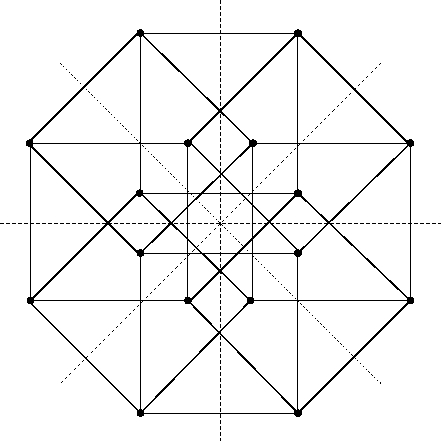and the vertices of a 4-dimensional orthoplex, namely $(\pm 2, 0, 0 , 0)$ and permutations: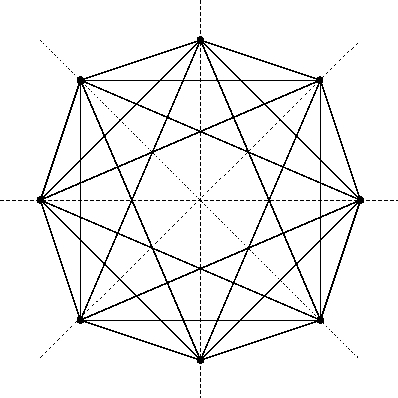and together they form the vertices of a 24-cell: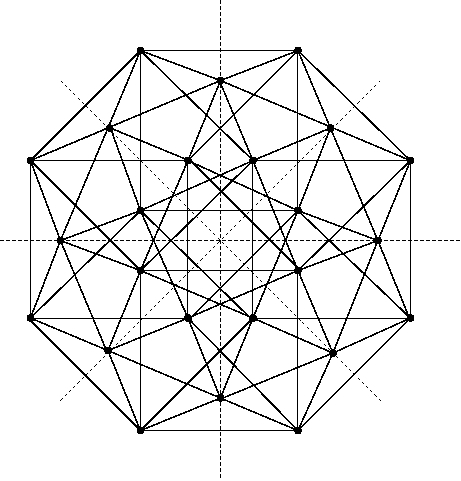(In case you forgot, an orthoplex or cross-polytope is the $n$-dimensional analogue of an octahedron, a regular polytope with $2n$ vertices.)

However, we can go further! If you take every other vertex of an $n$-dimensional cube — that is, start with one vertex and then all its second nearest neighbors and their second nearest neighbors and so on — you get the vertices of something called the $n$-dimensional demicube. In 3 dimensions, a demicube is a regular tetrahedron, so you can fit two tetrahedra in a cube like this:But in 4 dimensions, a demicube is an orthoplex! This is easy to see: if we take these points

$(\pm 1, \pm 1, \pm 1, \pm 1)$

and keep only those with an even number of minus signs, we get

$\pm (1,1,1,1)$

$\pm (1,1,-1,-1)$

$\pm (1,-1,1,-1)$

$\pm (1,-1,-1,1)$

which are the 8 vertices of an orthoplex.

So, we can take the vertices of a 24-cell and partition them into three 8-element sets, each being the vertices of an orthoplex!

And this has a nice representation-theoretic significance:

• the vertices of the first orthoplex correspond to the weights of the 8-dimensional vector representation of $\mathfrak{so}(8)$;

• the vertices of the second correspond to the weights of the 8-dimensional left-handed spinor representation of $\mathfrak{so}(8)$;

• the vertices of the third correspond to the weights of the 8-dimensional right-handed spinor representation of $\mathfrak{so}(8).$

Here I’m being a bit sneaky, identifying the weight lattice of $\mathrm{D}_4$ with the root lattice. In fact it’s twice as dense, but it ‘looks the same’: it’s the same up to a rescaling and rotation. The weight lattice contains a 24-cell whose vertices are the weights of the vector, left- and right-handed spinor representations. But the root lattice, which is what I really had been talking about, contains a larger 24-cell whose vertices are the roots — that is, the nonzero weights of the adjoint representation of $\mathfrak{so}(8)$ on itself.

Now, you may wonder which is the ‘first’ orthoplex, which is the ‘second’ one and which is the ‘third’, but it doesn’t matter much since there’s a symmetry between them! This is triality. The group $\mathrm{S}_3$ acts on $\mathfrak{so}(8)$ as outer automorphisms, and thus on the weight lattice and root lattice. So, it acts as symmetries of the 24-cell — and it acts by permuting the 3 orthoplexes!

But here’s the cool thing that Manivel focuses on. Suppose we take the 24-cell whose vertices are the roots of $\mathrm{D}_4$. Any orthoplex in here consists of 4 pairs of opposite roots along 4 orthogonal axes. So, it gives a root system of type $\mathrm{A}_1 \times \mathrm{A}_1 \times \mathrm{A}_1 \times \mathrm{A}_1$. In other words, it picks out a copy of $\mathfrak{sl}(2,\mathbb{C}) \oplus \mathfrak{sl}(2,\mathbb{C}) \oplus \mathfrak{sl}(2,\mathbb{C}) \oplus \mathfrak{sl}(2,\mathbb{C})$ in $\mathfrak{so}(8,\mathbb{C})$.

This Lie subalgebra

$\mathfrak{sl}(2,\mathbb{C}) \oplus \mathfrak{sl}(2,\mathbb{C}) \oplus \mathfrak{sl}(2,\mathbb{C}) \oplus \mathfrak{sl}(2,\mathbb{C}) \; \subset \; \mathfrak{so}(8,\mathbb{C})$

acts on $\mathfrak{so}(8,\mathbb{C})$ by the adjoint action. It acts on itself, and it acts on the rest of $\mathfrak{so}(8,\mathbb{C})$ via the representation

$\mathbb{C}^2 \otimes \mathbb{C}^2 \otimes \mathbb{C}^2 \otimes \mathbb{C}^2$

where each copy of $\mathfrak{sl}(2,\mathbb{C})$ acts on just one factor of $\mathbb{C}^2$ (in the obvious way). The weights of this ‘rest of’ $\mathfrak{so}(8,\mathbb{C})$ form the vertices of a 4-dimensional cube.

So, we’re getting a nice vector space isomorphism

$\mathfrak{so}(8,\mathbb{C}) \;\; \cong \;\; \mathfrak{sl}(2,\mathbb{C}) \oplus \mathfrak{sl}(2,\mathbb{C}) \oplus \mathfrak{sl}(2,\mathbb{C}) \oplus \mathfrak{sl}(2,\mathbb{C})\; \; \oplus \;\; \mathbb{C}^2 \otimes \mathbb{C}^2 \otimes \mathbb{C}^2 \otimes \mathbb{C}^2$

which is based on how the vertices of a 24-cell can be partitioned into the vertices of an orthoplex and a cube. But in fact we’re getting 3 such isomorphisms, related by triality!

If we call the 4 copies of $\mathbb{C}^2$ here $V_1, V_2, V_3, V_4$, we can write

$\mathfrak{so}(8,\mathbb{C}) \;\; \cong \;\; \bigoplus_{i = 1}^4 \mathfrak{sl}(V_i) \; \oplus \; \bigotimes_{i = 1}^4 V_i$

Also, we can write the three 8-dimensional irreducible representations of $\mathfrak{so}(8,\mathbb{C})$ as

$(V_1 \otimes V_2) \oplus (V_3 \otimes V_4)$

$(V_1 \otimes V_3) \oplus (V_2 \otimes V_4)$

$(V_1 \otimes V_4) \oplus (V_2 \otimes V_3)$

Note that they come from the three ways of partitioning a 4-element set into two 3-element sets.

Manivel calls this description of $\mathfrak{so}(8,\mathbb{C})$ the four-ality description, but I prefer to speak of tetrality. How is tetrality related to triality? The Dynkin diagram of $\mathrm{D}_4$ has $S_3$ symmetry, which yields triality: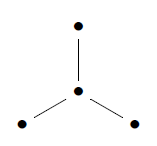But the corresponding extended or affine Dynkin diagram $\widetilde{\mathrm{D}}_4$ has $S_4$ symmetry, which yields tetrality: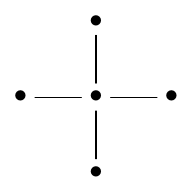One reason the affine Dynkin diagram is important is that maximal-rank Lie subalgebras of a Lie algebra with Dynkin diagram $X$ are obtained by deleting a dot from the corresponding affine Dynkin diagram $\widetilde{X}$. If we delete the middle dot of $\widetilde{\mathrm{D}}_4$ we get a disconnected diagram with 4 separate dots, which is the diagram for $\mathrm{A}_1 \times \mathrm{A}_1 \times \mathrm{A}_1 \times \mathrm{A}_1$. This is why $\mathfrak{sl}(2,\mathbb{C}) \oplus \mathfrak{sl}(2,\mathbb{C}) \oplus \mathfrak{sl}(2,\mathbb{C}) \oplus \mathfrak{sl}(2,\mathbb{C})$ shows up as a maximal rank Lie subalgebra of $\mathfrak{so}(8,\mathbb{C})$.

The relation between tetrality and triality, I believe, is that there’s a homomorphism $S_4 \to S_3$ coming from the 3 ways of partitioning a 4-element set into 2-element sets.

This is a good place to stop, though we’re really just getting started.

Posted at October 5, 2015 10:03 PM UTC

TrackBack URL for this Entry:   https://golem.ph.utexas.edu/cgi-bin/MT-3.0/dxy-tb.fcgi/2848

### Re: Configurations of Lines and Models of Lie Algebras (Part 2)

Just because the very-symmetric 24-cell left me scratching my head — not everything that looks like a square is a square.Posted by: Jesse C. McKeown on October 7, 2015 8:01 PM | Permalink | Reply to this

### Re: Configurations of Lines and Models of Lie Algebras (Part 2)

Posted by: John Baez on October 13, 2015 1:27 AM | Permalink | Reply to this

### Re: Configurations of Lines and Models of Lie Algebras (Part 2)

the three ways of partitioning a 4-element set into two 3-element sets.

do you mean 2 element sets?

Posted by: Charles G Waldman on October 8, 2015 12:11 AM | Permalink | Reply to this

### Re: Configurations of Lines and Models of Lie Algebras (Part 2)

Yes, sorry. That’s the famous trick that gives the homomorphism from $S_4$ to $S_3$. People know all the homomorphisms from $S_n$ to $S_m$ when $m \lt n$, and this is the most exciting one.

Posted by: John Baez on October 13, 2015 1:22 AM | Permalink | Reply to this

### Re: Configurations of Lines and Models of Lie Algebras (Part 2)

Thanks. I had been wondering if you were doing some sort of tropical categorified counting over the field with one element, where 3+3=4.

Just kidding (although it did confuse me for a few milliseconds). This is a really interesting paper, thanks for posting!

Posted by: Charles G Waldman on October 14, 2015 12:34 AM | Permalink | Reply to this

### Re: Configurations of Lines and Models of Lie Algebras (Part 2)

Let’s think about the last part over $\mathbb{F}_1$, and hence in the context of the $24$-cell.

There are two ways to get a $24$-cell from an orthoplex. One way, as the post says, is to take the orthoplex together with a scaled version of its dual hypercube, which consists of two demicubes, which are themselves orthoplexes in $4$ dimensions. The three orthoplexes together make up a $24$-cell.

The second way is to take the midpoints of the edges of the orthoplex. These give the vertices of another $24$-cell, dual to the first.

Let’s start by seeing how these things fit into the Dynkin diagram.

In any dimension, an orthoplex—or rather, the piebald orthoplex with alternately black and white highest-dimensional facets—is the polar space for $D_n$ (where $n$ is the dimension of the orthoplex). Vertices are isotropic points, edges are isotropic lines, faces isotropic planes, etc, of the orthogonal projective space of dimension $2n-1$ on which the orthogonal group (over $\mathbb{F}_1$) acts. The dots of the $D_n$ diagram, starting at the tip of the tail, correspond to the actions on points, on lines, etc. up to two types of subspace of maximal dimension $n$ at the “ears” of the diagram (except that there is no dot corresponding to the isotropic spaces of dimension $n-1$).

So the three outer points of $D_4$ correspond to a) the points of the polar space, i.e. the vertices of an $4$-orthoplex, and b) to the two kinds of highest-dimensional subspace of the polar space, i.e. the two kinds of facet (tetrahedra in this case) of the piebald orthoplex, whose centres lie at the vertices of two demicubes. So these, together, give one kind of $24$-cell.

And the central point of $D_4$ corresponds to the lines of the polar space, i.e. the edges of the orthoplex, and hence to the second kind of $24$-cell.

Now, given the second type of construction of a $24$-cell, we might wonder how to recover the three orthoplexes sitting inside it, in terms of the original orthoplex.

And the bit of the Manivel paper that the OP talks about does this for us!

Let’s translate the construction from $\mathbb{C}$ to $\mathbb{F}_1$.

Over $\mathbb{C}$ we have the subalgebra $\mathfrak{sl}(2,\mathbb{C}) \oplus \mathfrak{sl}(2,\mathbb{C}) \oplus \mathfrak{sl}(2,\mathbb{C}) \oplus \mathfrak{sl}(2,\mathbb{C})$, but for translating to the finite case, let’s go over to the group $SL(2,\mathbb{C})\times SL(2,\mathbb{C})\times SL(2,\mathbb{C})\times SL(2,\mathbb{C})$. Generally $SL(n,\mathbb{C})$ corresponds to the symmetric group $S_n$ over $\mathbb{F}_1$. So we have

$S_2\times S_2\times S_2\times S_2$

Now, just as $SL(2,\mathbb{C})$ has a natural action on $\mathbb{C}^2$, so $S_2$ has a natural action on a set with $2$ elements, say $\{-1,1\}$, which is the $\mathbb{F}_1$ analogue. And the direct sum of algebras acting on the tensor product of some vector spaces translates into a direct product of groups acting on the cartesian product of some sets, i.e. $S_2\times S_2\times S_2\times S_2$ acts on $\{-1,1\}\times\{-1,1\}\times\{-1,1\}\times\{-1,1\}$. So we can see clearly how a $4$-cube gets into the picture, with each factor of $S_2$ acting to flip the sign of one of the coordinates.

Now I need to say something about the edges of an orthoplex, but I’ll get there via a roundabout route.

It’s familiar that Euclidean rotations are basically “planar”; for instance, an arbitrary infinitesimal rotation can be decomposed as the sum of infinitesimal rotations in one or more mutually orthogonal planes, for instance those spanned by pairs of elements of an orthogonal basis. And this kind of carries over to other settings, such as the one we’re dealing with, where we have a very non-Euclidean orthogonal structure.

Over any field, in any space of even dimension, there is at least an orthogonal structure available under which the space can be decomposed as the orthogonal sum of hyperbolic planes. And each hyperbolic plane can be given a basis of two null vectors with a mutual orthogonal product of $1$. So we get a basis in which each basis element is orthogonal to all but one of the others. This is the sort of structure we’re thinking about here. Over $\mathbb{C}$ it’s the only sort there is.

We also get the same sort of structure over $\mathbb{F}_1$. Actually, once we’ve got the basis, we’ve got everything: the orthogonal polar space in $2n$ dimensions consists of $2n$ points, each orthogonal to all but one of the others, to which it is … not orthogonal. (You can’t really define an actual value for the dot product, but you can still distinguish orthogonal from non-orthogonal pairs of vectors/points.) We can see this in the orthoplex, where this feature of the orthogonal structure lifts to the containing Euclidean space: the vector pointing from the centre of the orthoplex to any of its vertices is orthogonal to all other such vectors except for one, viz. the one pointing to the diametrically opposite vertex.

Over an actual field, such as $\mathbb{C}$, we still get a basis of $\mathfrak{so}_{2n}$ coming from pairs of basis elements, which is why this algebra is $\left(\array{2n\\2}\right)$-dimensional, but $n$ of these pairs are different from the others because, unlike the others, they involve basis elements which are not mutually orthogonal. In fact, these different pairs will generate a Cartan subalgebra, and the $\mathbb{F}_1$ version of this stuff is how you go about constructing the roots, starting with this Cartan subalgebra.

More concretely, in an orthoplex, any pair of vertices which are not opposite are adjacent, so these non-opposite pairs are in bijection with edges of the orthoplex, which is how the roots of $\mathfrak{so}_{2n}$ come to be located at the midpoints of the edges of an orthoplex; which in $4$ dimensions form the vertices of a $24$-cell.

Now I can wrap up the final section of the post.

Writing one of the $8$-dimensional irreps of $\mathfrak{so}_8$ as, e.g. $(V_1 \otimes V_2) \oplus (V_3 \otimes V_4)$ corresponds, over $\mathbb{F}_1$, to decomposing the $8$ vertices of an orthoplex into the $4+4$ vertices of two mutually orthogonal squares. In this case, they would be $\left(\pm1,\pm1,0,0\right)$ and $\left(0,0,\pm1,\pm1\right)$. Clearly, these squares have vertices lying at the midpoints of the edges of our original orthoplex, and the $3$ ways of partitioning $4$ spaces into $2$ pairs give the $3$ ways to find a $4$-orthoplex in the $24$-cell whose vertices are the edge-midpoints of an $4$-orthoplex.

QED

Posted by: Tim Silverman on November 2, 2015 2:01 PM | Permalink | Reply to this

### Re: Configurations of Lines and Models of Lie Algebras (Part 2)

Brilliant! I was confused about the precise geometrical relation between the two 24-cells in my post:

The weight lattice contains a 24-cell whose vertices are the weights of the vector, left- and right-handed spinor representations. But the root lattice, which is what I really had been talking about, contains a larger 24-cell whose vertices are the roots — that is, the nonzero weights of the adjoint representation of $\mathfrak{so}(8)$ on itself.

But your comment explains that, among many other things.

I hadn’t known that the edge-midpoints of a 4-orthoplex form the vertices of a 24-cell!

Posted by: John Baez on November 2, 2015 4:05 PM | Permalink | Reply to this

### Re: Configurations of Lines and Models of Lie Algebras (Part 2)

I hadn’t known that the edge-midpoints of a 4-orthoplex form the vertices of a 24-cell!

I actually worked this out backwards, starting from the fact that the central dot of the $D_4$ diagram represents lines in the polar space and is invariant under triality, going, “I wonder if … ?” and then … umm … looking in Wikipedia—which has a couple of examples of coordinates for vertices of the $24$-cell, one of which makes this fact very clear once you know what you are looking for.

Took a while before I really integrated it with everything else, though.

Posted by: Tim Silverman on November 2, 2015 8:33 PM | Permalink | Reply to this

### Re: Configurations of Lines and Models of Lie Algebras (Part 2)

I had been a bit sad at first how little response this post garnered, but I realize now that I was just being impatient: it takes time to digest these ideas and do something interesting with them! Besides Tim’s new comment and Jesse’s picture, Layra Idarani has written a nice little paper:

which uses the ‘tetrality’ model of $\mathfrak{so}(8)$ described here to find a basis of this Lie algebra where the basis vectors are permuted by outer automorphisms of $\mathfrak{so}(8)$.

Layra also had some nice ideas about the three 8-dimensional irreps of $Spin(8)$:

Next thought: an outer automorphism that fixes the standard representation and swaps the spinor representations can be viewed as conjugation by a reflection in the standard representation. So the automorphism that swaps the standard rep with one of the spinor reps can be viewed as conjugation by a reflection in the other spinor rep.﻿

and

One benefit of having written this down is that it’s pretty clear that a rotation in a single plane in one representation does in fact become rotating at half the speed in four mutually orthogonal planes in the other reps, and not in a terribly confusing way […]

Posted by: John Baez on November 2, 2015 4:11 PM | Permalink | Reply to this

### Re: Configurations of Lines and Models of Lie Algebras (Part 2)

“I had been a bit sad at first how little response this post garnered”

Eventually there are less and less people who have the time to read what you write. Math is very time consuming.

“He also had some nice ideas about the three 8-dimensional irreps of Spin(8):”

Do you know “him” ?

I thought Layra was a female name: https://www.google.de/search?q=layra&source=lnms&tbm=isch&sa=X&ved=0CAcQAUoAWoVChMIpf–jbyyAIVyZtyCh22awCN&biw=1313&bih=767&dpr=0.9

### Re: Configurations of Lines and Models of Lie Algebras (Part 2)

Eventually there are less and less people who have the time to read what you write.

Well, 43 million people have looked at my posts on G+, so I don’t feel too neglected. But the post here was more technical. My goal was that anyone who understands semisimple Lie theory well should be able to understand it and enjoy it without much work. That’s a limited set of people. But once upon a time, when I was writing This Week’s Finds, I had a lot of online friends who knew that stuff well… so every time I wrote about it, they’d correct me and/or ask questions and/or add extra facts.

Unfortunately, those friends seem to have dispersed, and Lie theory is not so popular on the n-Café. Here, homotopy type theory is more popular! It’s equally technical, but more closely connected to the main goals of the n-Café. So that makes sense.

Math is very time consuming.

Mathematicians use that as an excuse not to do other things. It takes time to learn semisimple Lie theory, but it’s very popular topic among mathematicians, and once you know it, learning new facts about it is addictive as eating popcorn or playing go.

Do you know “him” ?

I actually don’t know Layra’s gender, and I don’t really need to know, so it was a mistake to randomly choose one. I’ll fix my comment. It’s annoying that English doesn’t have a singular neuter pronoun that’s grammatical for use with people… that’s what caused my mistake. Nowadays we use “they” or “them”, but I still don’t like it.

Posted by: John Baez on November 2, 2015 8:14 PM | Permalink | Reply to this

### Re: Configurations of Lines and Models of Lie Algebras (Part 2)

Shakespeare used “they”. The second example is plainly narration and not a character speaking in their specific voice.

And, of course, “you” is also a plural.

I wonder if mathematicians are less comfortable with numerical ambiguity than other sorts.

(there are at least two ways to parse that last sentence!)

Posted by: Jesse C. McKeown on November 3, 2015 6:31 PM | Permalink | Reply to this

### Re: Configurations of Lines and Models of Lie Algebras (Part 2)

I actually don’t know Layra’s gender, and I don’t really need to know, so it was a mistake to randomly choose one. I’ll fix my comment.

I didn’t know the name Layra either, before I looked it up via that image search (thats often what I do for looking up names). The ending “a” is however often connected with female names.

Layra Idarani seems to be a mock online identity, so Layra might still be a “he”.

I often try to find out a bit about authors.

Mathematicians use that as an excuse not to do other things. It takes time to learn semisimple Lie theory, but it’s very popular topic among mathematicians, and once you know it, learning new facts about it is addictive as eating popcorn or playing go.

It seems I am more a physicist, I am not so sure whether I am so thrilled by Lie theory per se. Representation theory seems important for elementary particle physics that’s why I once in a while try to have a quick look at it. But that’s now so rarely that I wouldn’t even call it a “hobby”.

Moreover “doing math” serves as an “excuse” basically only if you are getting paid for it.

I neither find eating popcorn or playing go addictive, but I think I understood what you wanted to say.

### Re: Configurations of Lines and Models of Lie Algebras (Part 2)

Ah, so my paper did make it here! Thanks for the share, John. It’s really just an explicit form of the construction in Landsberg and Manivel’s paper. I’m pretty sure that you can’t really make a better looking basis that is invariant under the $S_4$ action, but this one isn’t too terrible, I think. I still haven’t figured out what the order-3 automorphisms mean geometrically.

Re: gender Yeah, Layra’s not my real name, so analysis of it doesn’t really tell you much. I would start using my real name except after ten years of using the name online most people know me as Layra and it’s kind of my default identity, so it’s hard to switch. Oops. Anyway, don’t worry about pronouns; I don’t have strong feelings in any direction. I guess use “they” if it makes you more comfortable in the absence of further information.

Posted by: Layra on November 11, 2015 4:15 AM | Permalink | Reply to this

### Re: Configurations of Lines and Models of Lie Algebras (Part 2)

I had felt a bit guilty about taking so long to write this up! I had the ideas queued up waiting in my mind, but it took ages to work out a vaguely coherent sequence of words and paragraphs to express them.

Layra Idarani has written a nice little paper

This is fantastic, and makes me very happy. Lots to look at and think about.

Posted by: Tim Silverman on November 2, 2015 8:50 PM | Permalink | Reply to this

### Re: Configurations of Lines and Models of Lie Algebras (Part 2)

No problemo! I was not trying to guilt you for taking time to figure out this wonderful stuff! I was trying to guilt everyone else in the universe… well, everyone except Layra and Jesse.

(Until just now I disliked using ‘guilt’ as a transitive verb, because I thought it was a neologism and I’m a staunch traditionalist. But it turns out to go back to Middle English, and it’s pretty efficient.)

Posted by: John Baez on November 2, 2015 8:56 PM | Permalink | Reply to this

### Re: Configurations of Lines and Models of Lie Algebras (Part 2)

using ‘guilt’ as a transitive verb […] turns out to go back to Middle English

So does ‘they’ for a person of unknown gender!

Posted by: Tim Silverman on November 2, 2015 9:42 PM | Permalink | Reply to this

### Re: Configurations of Lines and Models of Lie Algebras (Part 2)

Posted by: John Baez on November 2, 2015 9:48 PM | Permalink | Reply to this

### Re: Configurations of Lines and Models of Lie Algebras (Part 2)

I managed to overlook this post when it first appeared, but in the time since then, I’ve discovered that this cluster of topics (the 24-cell, octonionic integers, the 27 lines on a cubic and the 28 bitangents to a quartic, etc.) is related to one of my research problems. So, thanks to the delay, I have the motivation to read it carefully!

Posted by: Blake Stacey on April 30, 2017 9:32 PM | Permalink | Reply to this

### Re: Configurations of Lines and Models of Lie Algebras (Part 2)

Thanks for telling us about that nice stuff!

Posted by: Thomas on November 7, 2015 8:20 PM | Permalink | Reply to this

### Re: Configurations of Lines and Models of Lie Algebras (Part 2)

This may be a silly question, but how does $\mathfrak{so}(8,\mathbb{C})$ act on $(V_1\otimes V_2)\oplus(V_3\otimes V_4)$? A priori it only looks like a rep of $\mathfrak{sl}(V_1)\oplus\mathfrak{sl}(V_2)\oplus\mathfrak{sl}(V_3)\oplus\mathfrak{sl}(V_4)$ to me…

Posted by: anon on April 18, 2017 8:38 PM | Permalink | Reply to this

### Re: Configurations of Lines and Models of Lie Algebras (Part 2)

Not sure if this is a priori or a posteriori but the vector representation of $SO(4)$ can be thought of as the representation $V_1 \otimes V_2$ of $SL(2) \times SL(2)$ and the vector representation of $SO(8)$ reduces as a direct sum in the representation $SO(4) \times SO(4)$

Posted by: William Schlieper on May 7, 2017 5:50 AM | Permalink | Reply to this

### Re: Configurations of Lines and Models of Lie Algebras (Part 2)

Manivel only says that when we restrict the three 8-dimensional irreps of $\mathfrak{so}(8,\mathbb{C})$ to the Lie subalgebra

$\mathfrak{sl}(2,\mathbb{C}) \oplus \mathfrak{sl}(2,\mathbb{C}) \oplus \mathfrak{sl}(2,\mathbb{C}) \oplus \mathfrak{sl}(2,\mathbb{C}) \; \subset \; \mathfrak{so}(8,\mathbb{C})$

we get representations equivalent to

$(V_1 \otimes V_2) \oplus (V_3 \otimes V_4)$

$(V_1 \otimes V_3) \oplus (V_2 \otimes V_4)$

$(V_1 \otimes V_4) \oplus (V_2 \otimes V_3)$

where $V_1, \dots , V_4$ are the two-dimensional irreps of the four copies of $\mathfrak{sl}(2,\mathbb{C})$.

Of course there must be some formulas for how all of $\mathfrak{so}(\mathbb{C})$ acts on these three spaces, but I don’t know them.